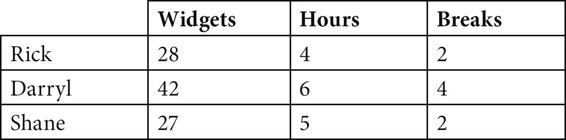# SAT Math Multiple Choice Question 234: Answer and Explanation

### Test Information

Question: 234

9.Rick, Shane, and Darryl work at a widget factory. The table above shows the number of hours they each spent at the factory on a given day, the number of widgets they produced, and the number of 15-minute breaks they took while they were at the factory. Each man works at a constant rate.

Rick and Shane are each assigned an equal number of widgets. Neither will take breaks in order to complete this assignment as quickly as possible. Rick offers to do a certain percentage of Shane's assignment so that they both finish at the same time. What percentage of Shane's original assignment does Rick do?

• A. 12.50%
• B. 14.30%
• C. 16.70%
• D. 25%